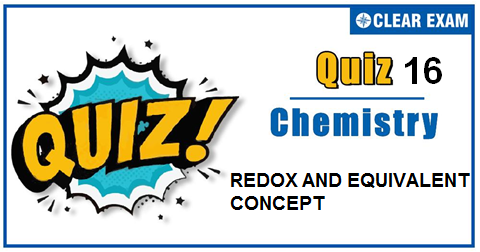## REDOX AND EQUIVALENT CONCEPT QUIZ-16

The one subject in NEET which is candidates who can easily attain good marks is Chemistry. That's the reason, often, one doesn't pay notice and choose to compromise it. But if one wants to rank above others, the tip is to be thorough with NEET chemistry concepts. The understanding of reactions and definite basic understanding is what requires major attention in Chemistry but once done it only gets simpler from there. The main focus on the to-do list should be on getting a hang of the NCERT syllabus of NEET chemistry. .

Q1. Weight of FeSO4 (mol.wt.=152) oxidized by 200 mL of 1 N KMnO4 solution is :
•  30.4 g
•  15.2 g
•  60.8 g
•  158 g
Solution
Meq.of FeSO4=Meq.of KMnO4 w/(152/1)× 1000=200 × 1 ∴w=30.4 g

Q2.Oxidation state of chromium
•  +10
•  +6
•  +3
•  +2
Solution

It is chromium peroxide. Let the oxidation number of Cr is ''x''. Cr(x+)+O2-+O(2-)+O2--CrO5 x+(-1)2+(-1)2+(-2)1=0 x-6=0 x=+6 Hence, the oxidation state of Cr is +6.

Q3.  What volume of 0.1 N oxalic acid solution can be reduced by 250 g of an 8 per cent by weight KMnO4 solution?
•   6.3 litre
•  12.6 litre
•  25.2 litre
•  0.63 litre
Solution
Meq.of oxalic acid=Meq.of KMnO4 V × 0.1 (250 × 8)/(100 × 31.6) × 1000=6.3 litre

Q4. The number of mole of KMnO4 that will be needed to react completely with one mole of ferrous oxalate in acidic solution is :
•  3/5
•  2/5
•  4/5
•  1
Solution
Mn(7+)+5e ⟶Mn(2+) Fe(2+)⟶Fe(3+ )+e C2(3+ ) ⟶2C(4+)+2e

Q5.A substance, that by its sharp colour change indicates the completion of reaction is known as :
•  Acid
•  Base
•  Indicator
•  None of these
Solution
Indicators are the substances which indicates the completion of a reaction.

Q6. The oxidation state of iron in sodium nitroprusside is :
•  +2
•  +1
• Zero
•  +3
Solution
Na2 [Fe(CN)5 NO]

Q7. Oxidation of thiosulphate (S2 O3(2-)) ions by iodine gives:
•  SO3-
•  SO4(2-)
•  S4 O6(2-)
•  S2 O8(2-)
Solution
2S2 O3(2-)+I2 ⟶S4 O6(2-)+2I-

Q8.What volume of 2N K2 Cr2 O7 solution is required to oxidise 0.81 g of H2 S in acidic medium?
•  47.8 mL
•  T23.8 mL
•  40 mL
•  72 mL
Solution
Meq.of K2 Cr2 O7=Meq.of H2 S 2 x V=0.81/(34/2) x 1000 ∴V=23.8 mL

Q9.The oxidation number of oxygen in KO3,Na2 O2 is
•  3,2
•  1,0
•  0,1
•  -0.33,-1
Solution
KO3 Na2 O2 Suppose O.N. of O = x suppose O.N. of O = x +1+3x=0 2×1+2x=0 3x= -1 2+2x= 0 x=-1/3 2x=-2 x=-0.33 x=-2/2 x= -1

Q10. Elements which generally exhibit multiple oxidation states and whose ions are coloured are known as :
•  Metalloid
•  Non-metals
•  Metals
• Transition metals
Solution
A characteristic property of transition elements.## Want to know more

Please fill in the details below:

## Latest NEET Articles\$type=three\$c=3\$author=hide\$comment=hide\$rm=hide\$date=hide\$snippet=hide

Name

ltr
item
BEST NEET COACHING CENTER | BEST IIT JEE COACHING INSTITUTE | BEST NEET & IIT JEE COACHING: REDOX AND EQUIVALENT CONCEPT QUIZ-16
REDOX AND EQUIVALENT CONCEPT QUIZ-16